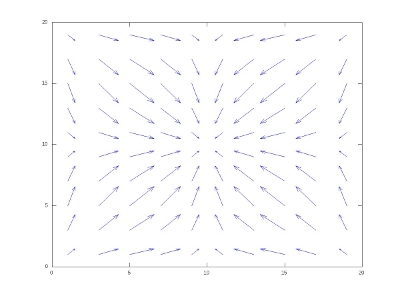Piecewise Deterministic Markov Processes (PDMP) constitute a very natural class of non-diffusion stochastic processes popularized by Mark H. A. Davis (he is now doing mainly mathematical finance). A PDMP ${(X_t)_{t\geq0}}$ with state space ${E}$ is a stochastic process built from three ingredients:

• Deterministic flow ${\Phi:E\times\mathbb{R}_+\rightarrow E}$
• Delay field ${\lambda:E\rightarrow\mathbb{R}_+}$
• Jump Kernel ${K:E\times E\rightarrow[0,1]}$

Starting from ${X_0=x}$, the process follows the deterministic flow up to the random time ${T_1}$ such that

$\int_0^{T_1}\!\lambda(\Phi(x,s))\,ds=E_1\sim\mathcal{E}(1).$

Then it jumps to state ${y}$ with law ${K(\Phi(x,T_1),\cdot)}$. The same mechanism is then used starting from ${y}$, etc. This explicit sample paths construction allows to simulate the process. If the deterministic flows have infinitesimal form ${G}$, then the infinitesimal generator of the process ${(X_t)_{t\geq0}}$ takes the transport-jump form

$(Lf)(x) = (Gf)(x)+\lambda(x)\int\!(f(y)-f(x))K(x,dy).$

This class of stochastic processes is more general than compound Poisson processes and basic queues, and includes also jump processes over vector fields. It appears in numerous modelling situations, such as in biology (cellular mass), physics (polymers length), computer science (TCP window size), reliability (workload and repairable systems), finance.

Bertrand Cloez will start his PhD (2010-2013) on these subjects. Here is a list of natural questions:

• Non-explosion ${T_\infty=\infty}$ a.s. and ergodicity ${X_t\rightarrow\mu}$ (see e.g. Last and Costa and Dufour)
• Speed of convergence ${d(X_t,\mu)\leq\varphi(X_0,t)}$ (see e.g. Fontbona, Guérin, Malrieu)
• Forward and backward couplings and perfect simulation
• Long time behavior of additive functionals ${t^{-1}\int_0^tf(X_s)\,ds}$
• Statistical estimation of the ingredients ${\Phi}$, ${\lambda}$, ${K}$
• Optimal stopping and control (see e.g. Goreac)
• Equilibrium regularity by mean of Hörmander type conditions
• Branching and transport-fragmentation PDEs (see e.g. Perthame)
• Links with growth-fragmentation (see e.g. Bertoin)
• Links with genealogical limit theorems (see e.g. Bansaye, Delmas, and Tran)

Last Updated on

This site uses Akismet to reduce spam. Learn how your comment data is processed.

Syntax · Style · .